International
Tables for
Crystallography
Volume A
Space-group symmetry
Edited by Th. Hahn

International Tables for Crystallography (2006). Vol. A. ch. 8.1, pp. 722-723

## Section 8.1.4. Crystal patterns, vector lattices and point lattices

H. Wondratscheka*

aInstitut für Kristallographie, Universität, D-76128 Karlsruhe, Germany
Correspondence e-mail: hans.wondratschek@physik.uni-karlsruhe.de

### 8.1.4. Crystal patterns, vector lattices and point lattices

| top | pdf |

Crystals are finite real objects in physical space which may be idealized by infinite three-dimensional periodic crystal structures' in point space. Three-dimensional periodicity means that there are translations among the symmetry operations of the object with the translation vectors spanning a three-dimensional space. Extending this concept of crystal structure to more general periodic objects and to n-dimensional space, one obtains the following definition:

Definition: An object in n-dimensional point spaceis called an n-dimensional crystallographic pattern or, for short, crystal pattern if among its symmetry operations

 (i) there are n translations, the translation vectors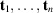of which are linearly independent, (ii) all translation vectors, except the zero vector o, have a length of at least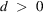.

Condition (i) guarantees the n-dimensional periodicity and thus excludes subperiodic symmetries like layer groups, rod groups and frieze groups. Condition (ii) takes into account the finite size of atoms in actual crystals.

Successive application of two translations of a crystal pattern results in another translation, the translation vector of which is the (vector) sum of the original translation vectors. Consequently, in addition to the n linearly independent translation vectors, all (infinitely many) vectors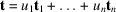(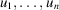arbitrary integers) are translation vectors of the pattern. Thus, infinitely many translations belong to each crystal pattern. The periodicity of crystal patterns is represented by their lattices. It is useful to distinguish two kinds of lattices: vector lattices and point lattices. This distinction corresponds to that between vector space and point space, discussed above. The vector lattice is treated first.

Definition: The (infinite) set of all translation vectors of a crystal pattern is called the lattice of translation vectors or the vector lattice L of this crystal pattern.

In principle, any set of n linearly independent vectors may be used as a basis of the vector space. Most of these sets, however, result in a rather complicated description of a given vector lattice. The following theorem shows that among the (infinitely many) possible bases of the vector spacespecial bases always exist, referred to which the survey of a given vector lattice becomes particularly simple.

Definitions: (1) A basis of n vectors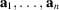ofis called a crystallographic basis of the n-dimensional vector lattice L if every integral linear combination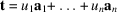is a lattice vector of L. (2) A basis is called a primitive crystallographic basis of L or, for short, a primitive basis if it is a crystallographic basis and if, furthermore, every lattice vector t of L may be obtained as an integral linear combination of the basis vectors.

The distinction between these two kinds of bases can be expressed as follows. Referred to a crystallographic basis, the coefficients of each lattice vector must be either integral or rational. Referred to a primitive crystallographic basis, only integral coefficients occur. It should be noted that nonprimitive crystallographic bases are used conventionally for the description of centred lattices', whereas reduced bases are always primitive; see Chapter 9.2.

#### Example

The basis used conventionally for the description of the cubic body-centred lattice' is a crystallographic basis because the basis vectors a, b, c are lattice vectors. It is not a primitive basis because lattice vectors with non-integral but rational coefficients exist, e.g. the vector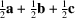. The bases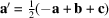,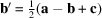,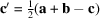or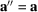,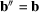,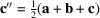are primitive bases. In the first of these bases, the vectoris given by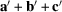, in the second basis by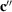, both with integral coefficients only.

Fundamental theorem on vector lattices: For every vector lattice L primitive bases exist.

It can be shown that (in dimensions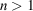) the number of primitive bases for each vector lattice is infinite. There exists, however, a procedure called basis reduction' (cf. Chapter 9.2), which uniquely selects one primitive basis from this infinite set, thus permitting unambiguous description and comparison of vector lattices. Although such a reduced primitive basis always can be selected, in many cases conventional coordinate systems are chosen with nonprimitive rather than primitive crystallographic bases. The reasons are given in Section 8.3.1. The term primitive' is used not only for bases of lattices but also with respect to the lattices themselves, as in the crystallographic literature a vector lattice is frequently called primitive if its conventional basis is primitive.

With the help of the vector lattices defined above, the concept of point lattices will be introduced.

Definition: Given an arbitrary point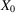in point space and a vector lattice L consisting of vectors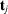, the set of all points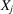with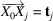is called the point lattice belonging toand L.

A point lattice can be visualized as the set of end-points of all vectors of L, where L is attached to an arbitrary pointof point space. Because each point X of point space could be chosen as the point, an infinite set of point lattices belongs to each vector lattice. Frequently, the pointis chosen as the origin of the coordinate system of the point space.

An important aspect of a lattice is its unit cell.

Definition: Ifis a crystallographic basis of a vector lattice L, the set of all vectorswith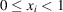is called a unit cell of the vector lattice.

The concept of a unit cell' is not only applied to vector lattices in vector space but also more often to crystal structures or crystal patterns in point space. Here the coordinate system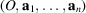and the originof the unit cell have to be chosen. In most cases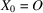is taken, but in general we have the following definition:

Definition: Given a crystallographic coordinate systemof a crystal pattern and a pointwith coordinates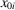, a unit cell of the crystal pattern is the set of all points X with coordinatessuch that the equation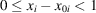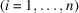holds.

Obviously, the term unit cell' may be transferred to real crystals. As the volume of the unit cell and the volumes of atoms are both finite, only a finite number N of atoms can occur in a unit cell of a crystal. A crystal structure, therefore, may be described in two ways:

 (a) One starts with an arbitrary unit cell and builds up the whole crystal structure by infinite repetition of this unit cell. The crystal structure thus consists of an infinite number of finite building blocks', each building block being a unit cell. (b) One starts with a point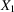representing the centre of an atom. To this point belong an infinite number of translationally equivalent points, i.e. points for which the vectors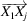are lattice vectors. In this way, from each of the points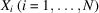within the unit cell a point lattice of translationally equivalent points is obtained. The crystal structure is then described by a finite number of interpenetrating infinite point lattices.

In most cases, one is not interested in the orientation of the vector lattice or the point lattices of a crystal structure in space, but only in the shape and size of a unit cell. From this point of view, a three-dimensional lattice is fully described by the lengths a, b and c of the basis vectors a, b and c and by the three interaxial angles α, β and γ. These data are called the lattice parameters, cell parameters or lattice constants of both the vector lattice and the associated point lattices of the crystal structure.